Next: The code Up: The Theory of General Previous: The Kerr metric

# Geodesics

We want to be able to calculate the trajectory of a freely falling object (massive or mass less). In special relativity, and in a local intertial frame, a freely falling object moves in a straight line / has a constant velocity. From there, we can go to general coodinates by using the ``trick'' of changing from an ordinary derivative to a covariant derivative. This gives the following equation of motion (also known as the geodesics equation):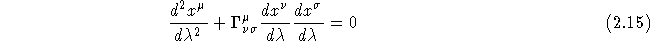, the affine parameter, is a parameter along the curve, which is unique up to a transformation of the form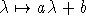(Misner, Thorne, & Wheeler 1973).

A light ray, which I want to study for this project, moves with the speed of light, which is the same as it has a null separation, i.e.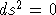. If we have a metric like the Kerr one and solve for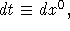we get a 2nd order equation: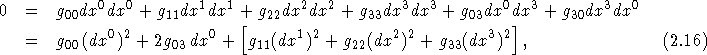where we have used the symmetry of the metric tensor, i.e.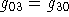. This equation can be readily solved. Notationwise, we could just as well have used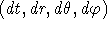instead of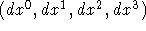.

Web Exhibition: Null Geodesics Around a Kerr Black HoleBo Milvang-Jensen (milvang@astro.ku.dk)
Mon Jun 17 11:54:08 MDT 1996